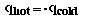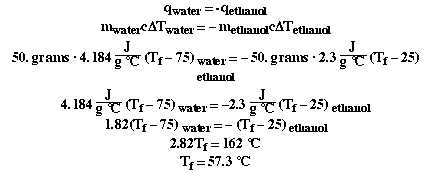### Mathematically we express that as;### This equation relates the amount of heat gained or lost by a sample of water in terms of the mass of the sample of water, its specific heat and the change in temperature the sample undergoes. So we can substitute that into our first equation (1st law of thermo);### So how do we handle using a different substance? We do the problem the exact same way. On the PLE we used ethyl alcohol. When 50. grams of water at 75 degrees Celsius is mixed with 50. grams of ethyl alcohol at 25 degrees Celsius, what is the final temperature, assuming no heat is lost to the surrounding?